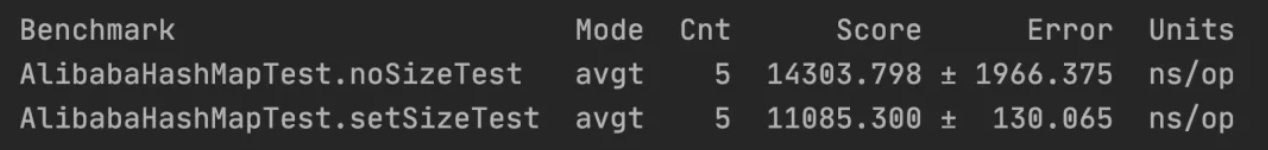# 阿里巴巴为什么让初始化集合时必须指定大小？

【推荐】集合初始化时，指定集合初始值大小。

## 规范解读

// 源码基于 JDK 8
final Node<K,V>[] resize() {
// 扩容前的数组
Node<K,V>[] oldTab = table;
// 扩容前的数组的大小和阈值
int oldCap = (oldTab == null) ? 0 : oldTab.length;
int oldThr = threshold;
// 预定义新数组的大小和阈值
int newCap, newThr = 0;
if (oldCap > 0) {
// 超过最大值就不再扩容了
if (oldCap >= MAXIMUM_CAPACITY) {
threshold = Integer.MAX_VALUE;
return oldTab;
}
// 扩大容量为当前容量的两倍，但不能超过 MAXIMUM_CAPACITY
else if ((newCap = oldCap << 1) < MAXIMUM_CAPACITY &&
oldCap >= DEFAULT_INITIAL_CAPACITY)
newThr = oldThr << 1; // double threshold
}
// 当前数组没有数据，使用初始化的值
else if (oldThr > 0) // initial capacity was placed in threshold
newCap = oldThr;
else {               // zero initial threshold signifies using defaults
// 如果初始化的值为 0，则使用默认的初始化容量
newCap = DEFAULT_INITIAL_CAPACITY;
}
// 如果新的容量等于 0
if (newThr == 0) {
float ft = (float)newCap * loadFactor;
newThr = (newCap < MAXIMUM_CAPACITY && ft < (float)MAXIMUM_CAPACITY ?
(int)ft : Integer.MAX_VALUE);
}
threshold = newThr;
@SuppressWarnings({"rawtypes","unchecked"})
Node<K,V>[] newTab = (Node<K,V>[])new Node[newCap];
// 开始扩容，将新的容量赋值给 table
table = newTab;
// 原数据不为空，将原数据复制到新 table 中
if (oldTab != null) {
// 根据容量循环数组，复制非空元素到新 table
for (int j = 0; j < oldCap; ++j) {
Node<K,V> e;
if ((e = oldTab[j]) != null) {
oldTab[j] = null;
// 如果链表只有一个，则进行直接赋值
if (e.next == null)
newTab[e.hash & (newCap - 1)] = e;
else if (e instanceof TreeNode)
// 红黑树相关的操作
((TreeNode<K,V>)e).split(this, newTab, j, oldCap);
else { // preserve order
// 链表复制，JDK 1.8 扩容优化部分
Node<K,V> loHead = null, loTail = null;
Node<K,V> hiHead = null, hiTail = null;
Node<K,V> next;
do {
next = e.next;
// 原索引
if ((e.hash & oldCap) == 0) {
if (loTail == null)
else
loTail.next = e;
loTail = e;
}
// 原索引 + oldCap
else {
if (hiTail == null)
else
hiTail.next = e;
hiTail = e;
}
} while ((e = next) != null);
// 将原索引放到哈希桶中
if (loTail != null) {
loTail.next = null;
}
// 将原索引 + oldCap 放到哈希桶中
if (hiTail != null) {
hiTail.next = null;
}
}
}
}
}
return newTab;
}


## 性能评测

<!-- https://mvnrepository.com/artifact/org.openjdk.jmh/jmh-core -->
<dependency>
<groupId>org.openjdk.jmh</groupId>
<artifactId>jmh-core</artifactId>
<version>{version}</version>
</dependency>


import org.openjdk.jmh.annotations.*;
import org.openjdk.jmh.infra.Blackhole;
import org.openjdk.jmh.runner.Runner;
import org.openjdk.jmh.runner.RunnerException;
import org.openjdk.jmh.runner.options.Options;
import org.openjdk.jmh.runner.options.OptionsBuilder;

import java.util.HashMap;
import java.util.Map;
import java.util.concurrent.TimeUnit;

@BenchmarkMode(Mode.AverageTime) // 测试完成时间
@OutputTimeUnit(TimeUnit.NANOSECONDS)
@Warmup(iterations = 2, time = 1, timeUnit = TimeUnit.SECONDS) // 预热 2 轮，每次 1s
@Measurement(iterations = 5, time = 3, timeUnit = TimeUnit.SECONDS) // 测试 5 轮，每次 3s
@Fork(1) // fork 1 个线程
public class AlibabaHashMapTest {
public static void main(String[] args) throws RunnerException {
// 启动基准测试
Options opt = new OptionsBuilder()
.include(AlibabaHashMapTest.class.getSimpleName()) // 要导入的测试类
.build();
new Runner(opt).run(); // 执行测试
}

@Benchmark
public void noSizeTest(Blackhole blackhole) {
Map map = new HashMap();
for (int i = 0; i < 1024; i++) {
map.put(i, i);
}
// 为了避免 JIT 忽略未被使用的结果
blackhole.consume(map);
}

@Benchmark
public void setSizeTest(Blackhole blackhole) {
Map map = new HashMap(1367);
for (int i = 0; i < 1024; i++) {
map.put(i, i);
}
// 为了避免 JIT 忽略未被使用的结果
blackhole.consume(map);
}
}## 总结

posted @ 2020-05-29 12:30  Java中文社群  阅读(685)  评论(0编辑  收藏